IUPAC-IUB Commission on Biochemical Nomenclature (CBN)

Abbreviations and Symbols for the Description of the Conformation of Polypeptide Chains

Tentative Rules (1969)

Continued from 2. The Sequence Rules and Choice of Torsion Angle

Contents

Continued with 4. Side Chains
Rule 3. The Main Chain (or Polypeptide Backbone)

Bonds between main-chain atoms are denoted by the symbols of the two atoms terminating them, e.g., Ni-Ciα, Ciα-Ci, Ci-Ni+1, Ci-Oi, Ni-Hi. Abbreviated symbols should not be used. Bond lengths are written b(Ci,Ni+l), etc.

3.2.1. The principal torsion angle describing rotation about N-Cα is denoted by φ, that describing rotation about Cα-C is denoted by ψ, and that describing rotation about C-N is denoted by ω. The symbols φi, ψi, and ωi are used to denote torsion angles of bonds within the i th residue in the case of φ and ψ and between the i th and (i +1)th residues in the case of ω; specifically, φi refers to the torsion angle of the sequence of atoms Ci-1, Ni, Ciα, Ci; ψito the sequence Ni, Ciα, Ci, Ni+l; and ωi to the sequence Ciα, Ci, Ni+1, Ci+1α (see Figure 5). In accordance with Rules 1.6 and 2.1.1, these torsion angles are ascribed zero values for eclipsed conformations of the main-chain atoms N, Cα, and C, that is, for the so-called cis conformations (see Table I).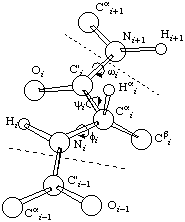Figure 5: Perspective drawing of a section of polypeptide chain representing two peptide units. The limits of a residue are indicated by dashed lines, and recommended notations for atoms and torsion angles are indicated. The chain is shown in a fully extended conformation (φi = ψi = ωi = 180°), and the residue illustrated is L.
Notes

(i) This convention differs from that proposed by Edsall et al. (1966). The new designation of angles may be derived from the old by adding 180° to, or subtracting 180° from, the latter. (This statement is precisely correct only if the peptide bond is exactly planar, which is not generally the case in experimentally determined structures).

(ii) Owing to the partial double-bond character of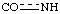, it is normally possible for ω to assume values only in the A neighborhood of 0 or 180°. ω ~ 180° is the value which is generally found (i.e., the trans conformation),

(iii) A "fully extended" polypeptide chain is characterized by φ = ψ = ω = +180°. The case of φ = ψ = 0° would involve the relations indicated in Table I.

(iv) Table II gives values of φ and ψ for various well-known regular structures. It is noteworthy that a right-handed α helix has negative torsion angles.

(v) Figure 6 is a typical conformational map ( φ-ψ plot) using the rules enunciated above.

Table I Main-Chain Torsion Angles for Various Conformations in Peptides of L-Amino Acids.a,b

0Cα-C trans0Cα-N trans
+60Cα-H cis+60Cα-R cis
+120Cα-R trans+120Cα-H trans
+180Cα-C cis+180Cα-N cis
-120Cα-H trans-120Cα-R trans
-60Cα-R cis-60Cα-H cis

a trans to Ni-Hi is the same as cis to Ni-Ci-1; trans to Ci-Oi is the same as cis to Ci-Ni+1, (see Figure 5).

b For the description of D-amino acids, interchange Cα-H and Cα-R in the table.

Table II: Approximate Torsion Angles for Some Regular Structures.a

φ (deg)ψ (deg)ω (deg)Reference
Right handed α helix (α-poly(L-alanine))-57-47+180Arnott and Dover (1967)
Left-handed α helix+57+47+180Arnott and Dover (1967)
Parallel-chain pleated sheet-119+113+180Schellman and Schellman (1964)
Antiparallel-chain pleated sheet (β-poly(L-alanine))-139+135-178Arnott et al. (1967)
Polyglycine II-80+150+180Ramachandran et al. (1966)
Collagen-51, -76, -45+153, +127, +148+180Yonath and Traub (1969)
Poly(L-proline) I-83+1580Ramachandran and Sasisekharan (1968),
calculated from
Traub and Schmueli (1963)
Poly(L-proline) II-78+149+180Arnott and Dover (1968)

a For a fully extended chain φ = ψ = &oega; = +180°.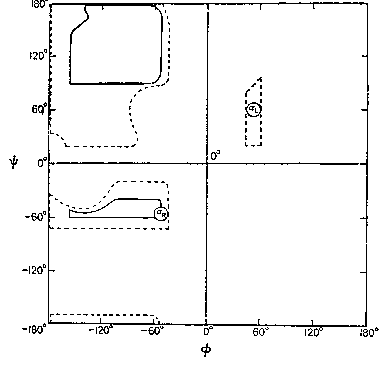Figure 6: Typical conformatial map (Ramachandran et al., 1963) transposed into the standard conventions. Note: this diagram is identical with that of Edsall et al. (1966) except that the origin is now at the center, instead of at the lower left hand corner. The solid lines enclose the freely allowed values of φ and ψ for an alanyl residue in a polypeptide; the dotted lines enclose "outer limit" values based on the shortest known van der Waals radii in related structures. Analogous diagrams for other residues, and for slightly different assumptions, are given by Ramachandran and Sasisekharan (1968; note that these authors used the earlier convention with the origin at the corner).
3.2.2. There may occasionally be a need to consider torsion angles differing from zero for the sequences of atoms O=C-N-Cα and Cα-C-N-H, in cases where C=O or N-H lies out of the peptide plane. These angles may be represented υO and υH (Greek upsilon).

3.3.1. If the terminal amino group of the chain is protonated the three hydrogen atoms are denoted H11, H12, and H13; the hydrogen atom giving the smallest (positive or negative) value of the principal torsion angle H-N-Cα-C is denoted H11 and the others are numbered in a clockwise sense when viewed in the direction Cα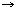N. The corresponding torsion angles are denoted φ11, φ12 and φ13. If the terminal amino group is not protonated the hydrogen atoms are denoted H11 and H12 in accordance with Rule 2.2.2, and the corresponding torsion angles, φ11 and φ12.

3.3.2. At the carboxyl terminus of the chain (i = T) the double-bonded oxygen is written as O' and the other oxygen as O", thus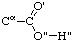The torsion angles about the Cα-C bond are written ψT1 and ψT2 (or ψ1(T), ψ2(T)); the torsion angle about the C-O" bond, defining the orientation of the hydrogen atom of the hydroxyl group relative to Cα, is written θTC (or θC(T)). If the terminal carboxyl group is ionized the oxygen atoms are denoted O' and O", the precedence being determined by Rule 2.3.2, and the torsion angles are written as before.

Note. Instead of O' and O" the alternative notations O1 and O2 may be used. ψT may be used instead of ψT1, in conformity with the convention for the middle of the chain, so long as confusion does not arise.

3.3.3. Substituted terminal groups. Natural extensions of the above rules may be devised, e.g.

(i) N-formyl group H0-C0O0-N1H1-C1αH1α-

(ii) N-acetyl group C0(H01,H02,H03)-C0O0-N1H1-C1αH1α-

(iii) C-amido group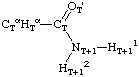References for this section

Arnott, S., and Dover, S. D. (1967), J. Mol. Biol. 30, 209.

Arnott, S., and Dover, S. D. (1968), Acta Cryst. B24, 599.

Arnott, S., Dover, S. D., and Elliott, A. (1967), J. Mol. Biol. 30, 201.

Edsall, J. T., Flory, P. J., Kendrew, J. C., Liquori, A. M., Némethy, G., Ramachandran, G. N., and Scheraga, H. A. (1966), J. Biol. Chem. 241, 1004; Biopolymers 4, 130; J. Mol. Biol. 15, 339.

Ramachandran, G. N., Ramakrishnan, C., and Sasisekharan, V. (1963), J. Mol. Biol. 7, 95.

Ramachandran, G. N., and Sasisekharan, V. (1968), Advan. Protein Chem. 23, 283.

Ramachandran, G. N., Sasisekharan, V., and Ramakrishnan, C. (1966), Biochim. Biophys. Acta 112, 168.

Schellman, J. A., and Schellman, C. (1964), Proteins 2, 1.

Traub, W., and Schmueli, U. (1963), in Aspects of Protein Structure, Ramachandran, G. N., Ed., Academic Press, London, p 81.

Yonath, A.,and Traub, W. (1969), J. Mol. Biol. 43, 461.

Continued with 4. Side Chains